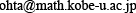Yasuhiro OHTA Yasuhiro OHTA Department of Mathematics, Graduate School of Science, Kobe University Division : Analysis Professor Building B, Room 310Research Field : Nonlinear Integrable System, Applied Mathematics, Mathematical Engineering Research Summary : I am working on nonlinear integrable systems, in particular, nonlinear evolution equations such as soliton equations and Painleve equations. Algebraic structure of solution space and properties of exact solutions are investigated. Their applications to fluid dynamics, optical communications, numerical analysis are also studied. For example we can regard numerical algorithm as dynamical system and the mathematical structure of the algorithm are analyzed from the study of evolution equations. Primary Publications : C. Gilson, J. Hietarinta, J. Nimmo and Y. Ohta, Sasa-Satsuma Higher-Order Nonlinear Schrödinger Equation and Its Bilinearization and Multisoliton Solutions, Phys. Rev. E, 68 (2003) 016614. Y. Ohta, Discretization of Toroidal Soliton Equations, J. Nonlinear Math. Phys., 10 Suppl. 2 (2003) 143--148. K. Kajiwara, T. Masuda, M. Noumi, Y. Ohta and Y. Yamada, Hypergeometric Solutions to the \$q\$-Painlevé Equations, Int. Math. Res. Not., 47 (2004) 2497--2521. Chun-Xia Li and Y. Ohta, Gram-Type Pfaffian Solution to the Coupled Discrete KP Equation, J. Phys. A, 38 (2005) 1089--1095. K. Kajiwara, T. Masuda, M. Noumi, Y. Ohta and Y. Yamada, Construction of Hypergeometric Solutions to the \$q\$-Painlevé Equations, Int. Math. Res. Not., 24 (2005) 1439--1463. K. Kajiwara, T. Masuda, M. Noumi, Y. Ohta and Y. Yamada, Cubic Pencils and Painlevé Hamiltonians, Funkcial. Ekvac., 48 (2005) 147--160. M. Nishizawa, Y. Ohta and S. Tsujimoto, Some Aspects of the Toda Molecule, Glasgow Math. J., 47A (2005) 169--176.